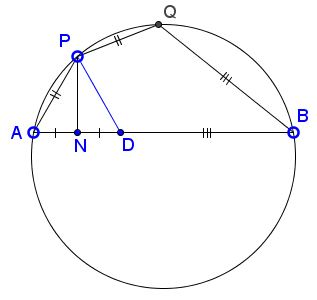# The Book of Lemmas: Proposition 3 What is this about? A Mathematical Droodle

2 January 2016, Created with GeoGebra

ExplanationThe applet attempts to suggest Proposition 3 from Archimedes' Book of Lemmas:

Let P be any point on a segment of a circle whose base is AB, and let PN be perpendicular to AB. Take D on AB so that AN = ND. If now PQ be an arc equal to the arc PA, and BQ be joined, then BQ, BD shall be equal.### Proof

Join PA, PQ, PD, DQ.Then, since the arcs PA, PQ are equal,

PA = PQ.

But, since AN = ND, and angles at N are right,

PA = PD.

Therefore PQ = PD and ∠PQD = ∠PDQ.

Now, since A, P, Q, B are concyclic,

∠PAD + ∠PQB = (two right angles),

whence

 ∠PDA + ∠PQB = (two right angles) = ∠PDA + ∠PDB.

Therefore

∠PQB = ∠PDB;

and, since the parts, the angles PQD, PDQ, are equal,

∠BQD = ∠BDQ,

and BQ = BD.### Archimedes' Book of Lemmas

1. Proposition 1: If two circles touch at A, and if CD, EF be parallel diameters in them, ADF is a straight line.

2. Proposition 2: Let AB be the diameter of a semicircle, and let the tangents to it at B and at any other point D on it meet in T. If now DE be drawn perpendicular to AB, and if AT, DE meet in F, then DF = FE..

3. Proposition 3: Let P be any point on a segment of a circle whose base is AB, and let PN be perpendicular to AB. Take D on AB so that AN = ND. If now PQ be an arc equal to the arc PA, and BQ be joined, then BQ, BD shall be equal.

4. Proposition 4: If AB be the diameter of a semicircle and N any point on AB, and if semicircles be described within the first semicircle and having AN, BN as diameters respectively, the figure included between the circumferences of the three semicircles is "what Archimedes called arbelos"; and its area is equal to the circle on PN as diameter, where PN is perpendicular to AB and meets the original semicircle in P.

5. Proposition 5: Let AB be the diameter of a semicircle, C any point on AB, and CD perpendicular to it, and let semicircles be described within the first semicircle and having AC, CB as diameters. Then if two circles be drawn touching CD on different sides and each touching two of the semicircles, the circles so drawn will be equal.

6. Proposition 6: Let AB, the diameter of a semicircle, be divided at C so that AC = 3/2·CB [or in any ratio]. Describe semicircles within the first semicircle and on AC, CB as diameters, and suppose a circle drawn touching the all three semicircles. If GH be the diameter of this circle, to find relation between GH and AB.

7. Proposition 7: If circles are circumscribed about and inscribed in a square, the circumscribed circle is double of the inscribed square.

8. Proposition 8: If AB be any chord of a circle whose centre is O, and if AB be produced to C so that BC is equal to the radius; if further CO meets the circle in D and be produced to meet the circle the second time in E, the arc AE will be equal to three times the arc BD.

9. Proposition 9: If in a circle two chords AB, CD which do not pass through the centre intersect at right angles, then (arc AD) + (arc CB) = (arc AC) + (arc DB).

10. Proposition 10: Suppose that TA, TB are two tangents to a circle, while TC cuts it. Let BD be the chord through B parallel to TC, and let AD meet TC in E. Then, if EH be drawn perpendicular to BD, it will bisect it in H.

11. Proposition 11: If two chords AB, CD in a circle intersect at right angles in a point O, not being the centre, then AO2 + BO2 + CO2 + DO2 = (diameter)2.

12. Proposition 12: If AB be the diameter of a semicircle, and TP, TQ the tangents to it from any point T, and if AQ, BP be joined meeting in R, then TR is perpendicular to AB.

13. Proposition 13: If a diameter AB of a circle meet any chord CD, not a diameter, in E, and if AM, BN be drawn perpendicular to CD, then CN = DM.

14. Proposition 14: Let ACB be a semicircle on AB as diameter, and let AD, BE be equal lengths measured along AB from A, B respectively. On AD, BE as diameters describe semicircles on the side towards C, and on DE as diameter a semicircle on the opposite side. Let the perpendicular to AB through O, the centre of the first semicircle, meet the opposite semicircles in C, F respectively. Then shall the area of the figure bounded by the circumferences of all the semicircles be equal to the area of the circle on CF as diameter.

15. Proposition 15: Let AB be the diameter of a circle., AC a side of an inscribed regular pentagon, D the middle point of the arc AC. Join CD and produce it to meet BA produced in E; join AC, DB meeting in F, and Draw FM perpendicular to AB. Then EM = (radius of circle).

### Reference

1. Great Books of The Western World, v. 11, Encyclopaedia Britannica, 1952